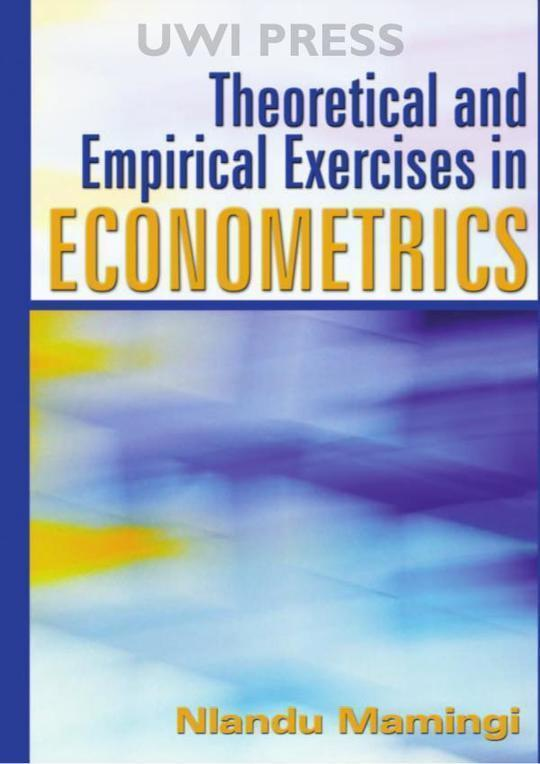\$29.99

# Theoretical and Empirical Exercises in Econometrics

By Nlandu Mamingi
US\$ 29.99
The publisher has enabled DRM protection, which means that you need to use the BookFusion iOS, Android or Web app to read this eBook. This eBook cannot be used outside of the BookFusion platform.
Book Description
• Contents
• Tables
• Figures
• Foreword
• Preface
• Acknowledgments
• Abbreviations and Symbols
• Part 1. Single Equation Regression Models
• 1. The Classical Linear Regression Model
• 2. Relaxation of Assumptions of the Classical Linear Model
• 3. Dummy Variables and Limited Dependent Variables
• Part 2. Simultaneous Equations Models
• 4. Simultaneous Equations Models
• Part 3. Dynamic Regression Models
• 5. Dynamic Regression Models
• 6. Unit Root, Vector Autoregressions, Cointegration and Error Correction Models
• 7. Aggregation Over Time
• Part 4. Other Topics
• 8. Forecasting
• 9. Panel Data Models
• References
• Index
The book hasn't received reviews yet.
You May Also Like